Question

2. For the pin-jointed truss shown in Figure Q2.1 applied at node 4. The Young's modulus E(GPa) is the same for...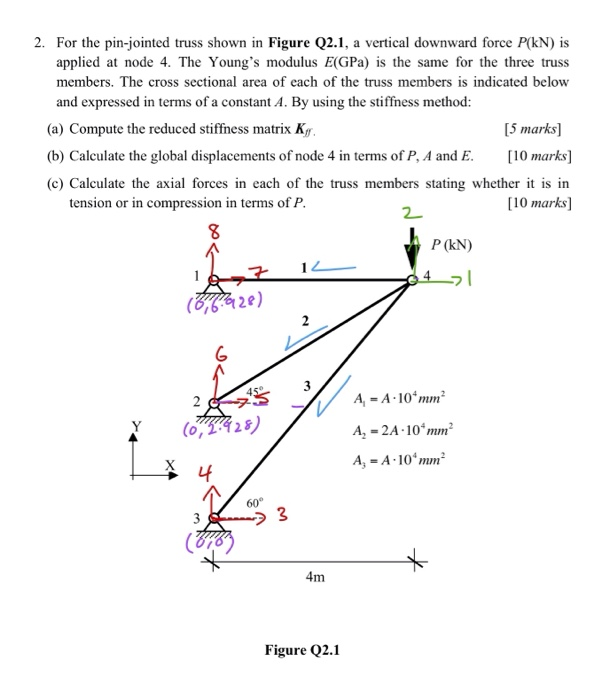2. For the pin-jointed truss shown in Figure Q2.1 applied at node 4. The Young's modulus E(GPa) is the same for the three truss vertical downward force P(kN) is a members. The cross sectional area of each of the truss members is indicated below and expressed in terms of a constant A. By using the stiffness method: (a) Compute the reduced stiffness matrix Kg [5 marks [10 marks (b) Calculate the global displacements of node 4 in terms of P, A and E (c) Calculate the axial forces in each of the truss members stating whether it is in tension or in compression in terms of P. [10 marks] 2 P (kN) (&ffz2e) G A 10 mm2 A (0428) A 2A-10 mm2 A, A 10 mm 60° 3 3 4m Figure Q2.1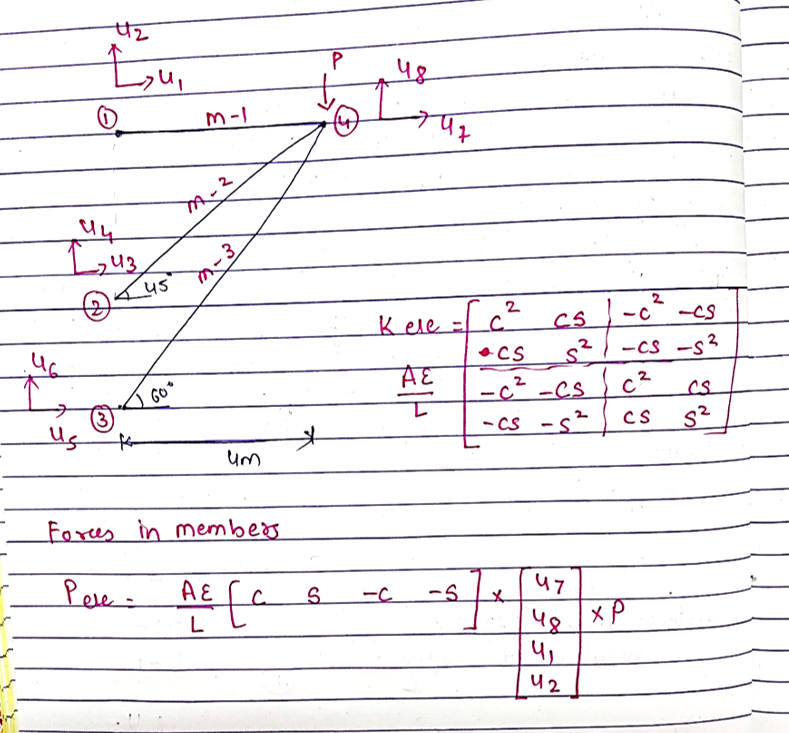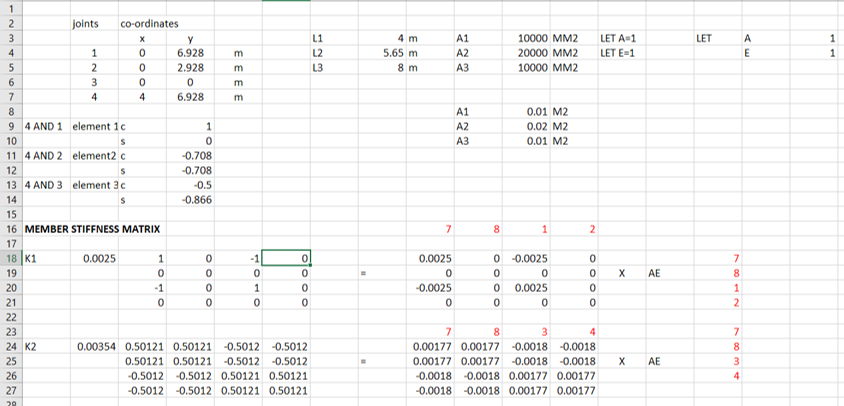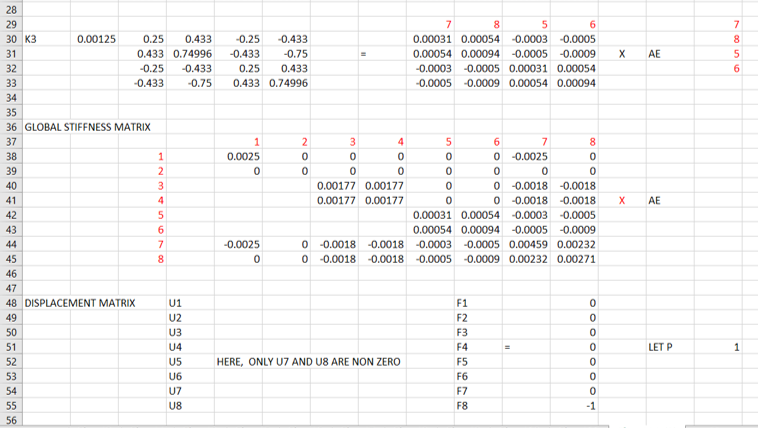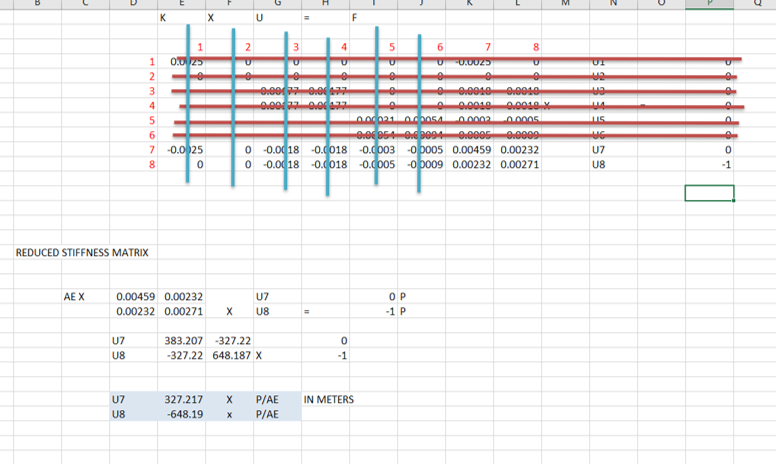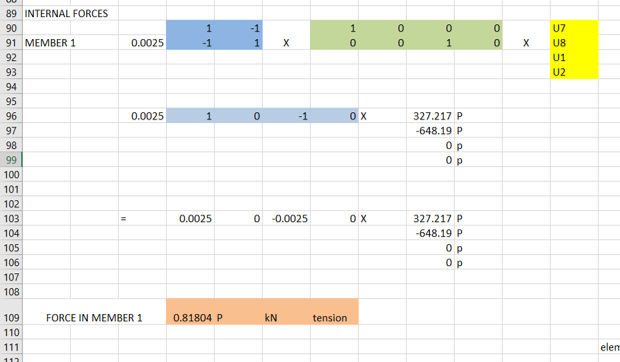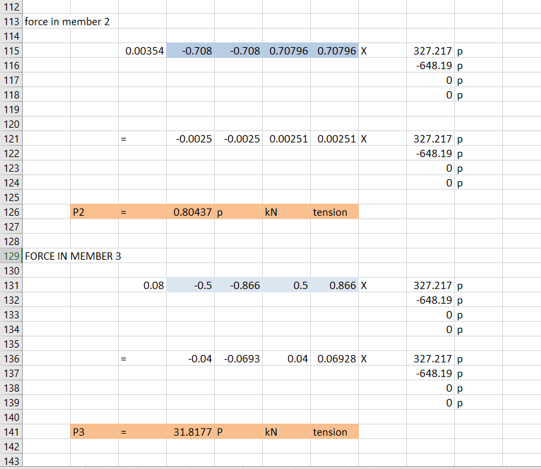Earn Coins

Coins can be redeemed for fabulous gifts.

Similar Homework Help Questions
• The plane truss is subjected to a load as shown in Figure 4. Take E = 200 GPa and cross sectional areas of members 1, 2...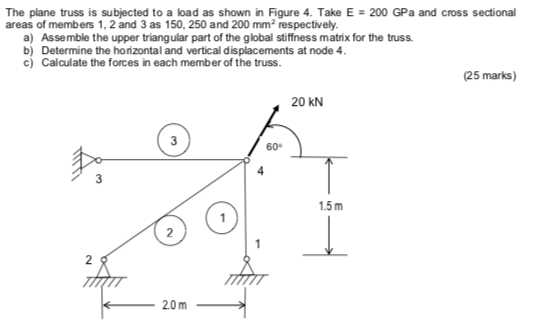The plane truss is subjected to a load as shown in Figure 4. Take E = 200 GPa and cross sectional areas of members 1, 2 and 3 as 150, 250 and 200 mm2 respectively a) Assemble the upper triangular part of the global stiffness matrix for the truss b) Determine the horizontal and vertical displacements at node 4 c) Calculate the forces in each member of the truss. (25 marks) 20 kN 3 60° 4 1.5m 2 2 20m...

• Question 4 The plane truss is subjected to a load as shown in Figure 4. Take E = 200 GPa and cross sectional areas of m...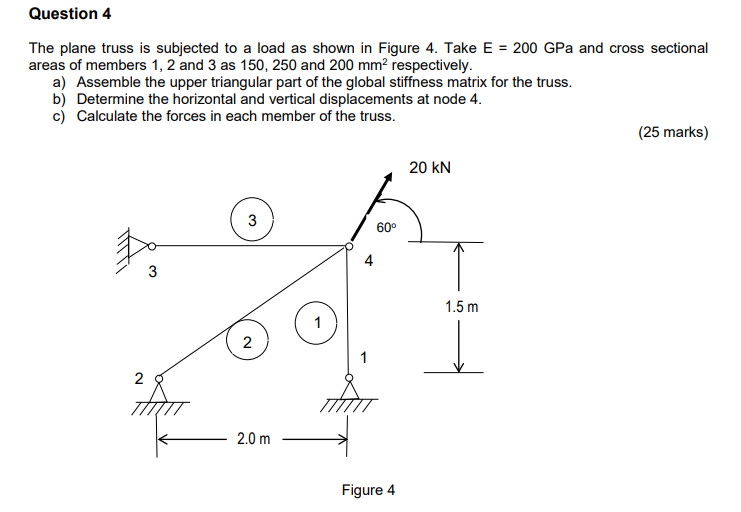Question 4 The plane truss is subjected to a load as shown in Figure 4. Take E = 200 GPa and cross sectional areas of members 1, 2 and 3 as 150, 250 and 200 mm2 respectively a) Assemble the upper triangular part of the global stiffness matrix for the truss. b) Determine the horizontal and vertical displacements at node 4. c) Calculate the forces in each member of the truss. (25 marks) 20 kN 3 600 4 3 1.5m...

• Please solve this question clearly and step by step. Thank you 2. A truss assembly shown...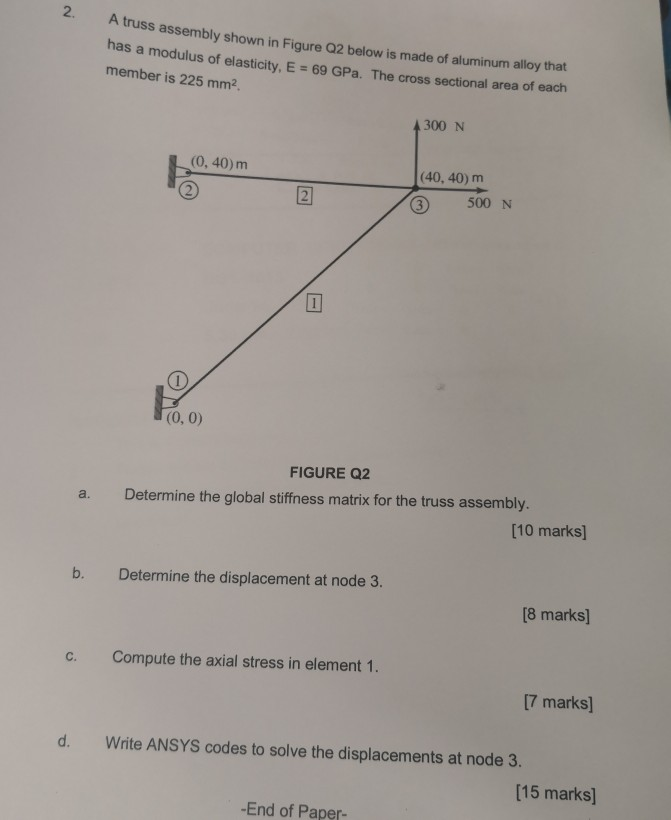Please solve this question clearly and step by step. Thank you 2. A truss assembly shown in Figure Q2 below is made of aluminum alloy that has a modulus of elasticity, E = 69 GPa. member is 225 mm2 The cross sectional area of each 4300 N (0, 40) m (40, 40) m 2 500 N 3 (0, 0) FIGURE Q2 Determine the global stiffness matrix for the truss assembly. a. [10 marks] Determine the displacement at node 3. b....

• Figure Q5(a) shows a plane truss supported by a horizontal spring at the top node. The...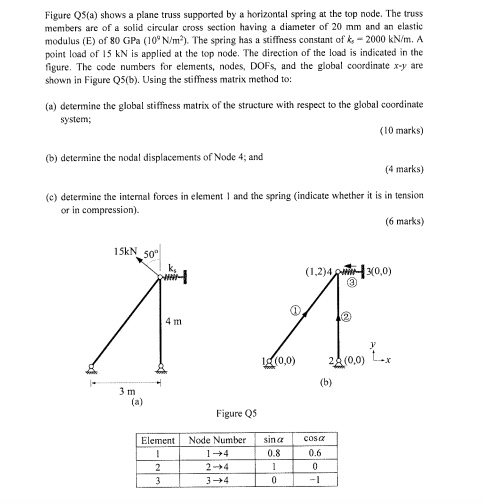Figure Q5(a) shows a plane truss supported by a horizontal spring at the top node. The truss members are of a solid circular cross section having a diameter of 20 mm and an elastic modulus (E) of 80 GPa (10° N/m2). The spring has a stiffness constant of k-2000 kN/m. A point load of 15 kN is applied at the top node. The direction of the load is indicated in the figure. The code numbers for elements, nodes, DOFS, and...

• Q1 The pin-jointed wood truss shown in Figure Q1a is subjected to a point load P...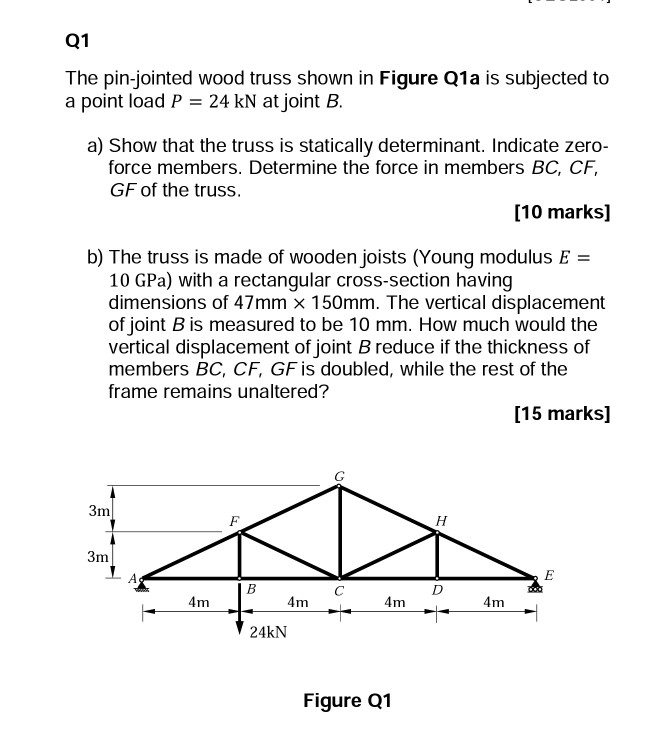Q1 The pin-jointed wood truss shown in Figure Q1a is subjected to a point load P 24 kN at joint B a) Show that the truss is statically determinant. Indicate zero force members. Determine the force in members BC, CF, GF of the truss [10 marks] b) The truss is made of wooden joists (Young modulus E 10 GPa) with a rectangular cross-section having dimensions of 47mm × 150mm. The vertical displacement of joint B is measured to be 10...

• please answer question 2a and 2b with clear steps and remarks (a) For the pin-jointed truss...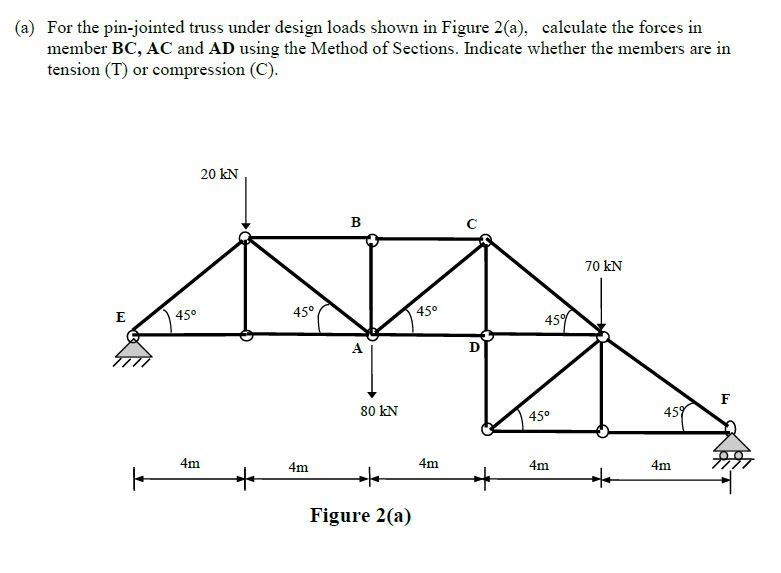please answer question 2a and 2b with clear steps and remarks (a) For the pin-jointed truss under design loads shown in Figure 2(a), member BC, AC and AD using the Method of Sections. Indicate whether the members are in tension (T) or compression (C) calculate the forces in 20 kN 70 kN 45° 45° 45° 45 80 kN 45° 45 4m 4m 4m L 4m 4m Figure 2(a) (b) A sample of a steel member was taken for tensile testing...

• Question Four: For the pin-jointed truss shown in Figure 4, 3m 12 KN 3m 30 kN 3m 15 kN 4m 4m 3m 3m 6m Figure 4. Calcula...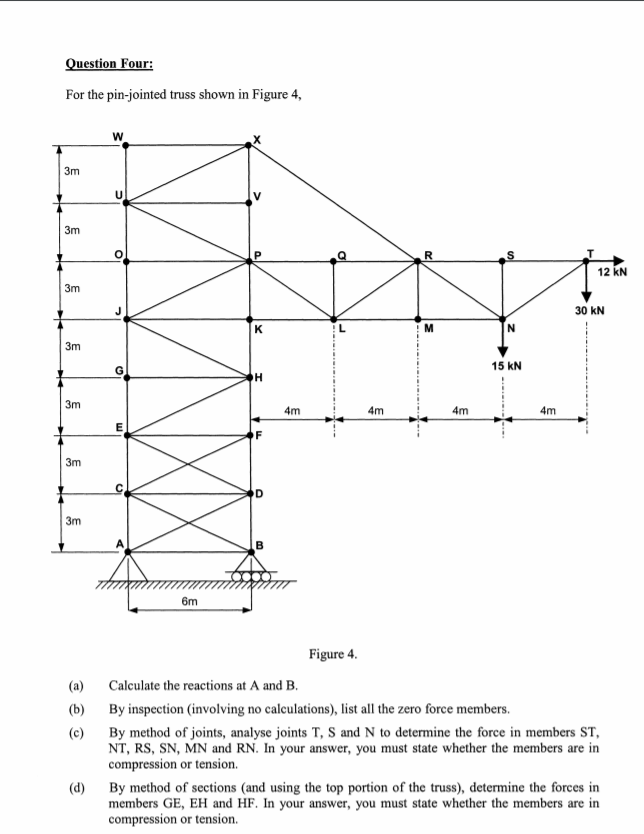Question Four: For the pin-jointed truss shown in Figure 4, 3m 12 KN 3m 30 kN 3m 15 kN 4m 4m 3m 3m 6m Figure 4. Calculate the reactions at A and B. (a) By inspection (involving no calculations), list all the zero force members. (b) (c) By method of joints, analyse joints T, S and N to determine the force in members ST NT, RS, SN, MN and RN. In your answer, you must state whether the members are...

• The plane truss shown in Figure is composed of members having a square 15 mm ×...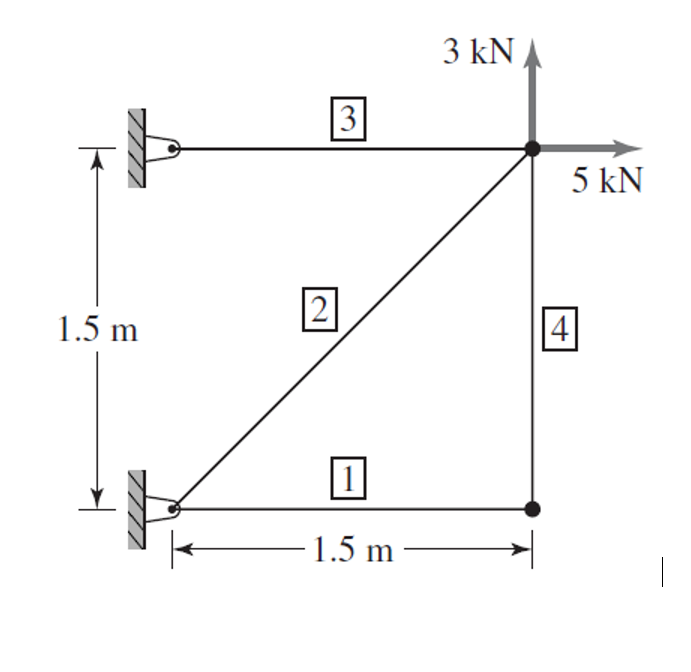The plane truss shown in Figure is composed of members having a square 15 mm × 15 mm cross section and modulus of elasticity E = 69 GPa. a. Assemble the global stiffness matrix. b. Compute the nodal displacements in the global coordinate system for theloads shown. c. Compute the axial stress in each element 3 kN 3 5 kN 2 1.5 m 4. 1.5 m

• 080 Solid Mechanics.pdf Download Print > Save to OneDrive Question 4 The pin-jointed truss shown in...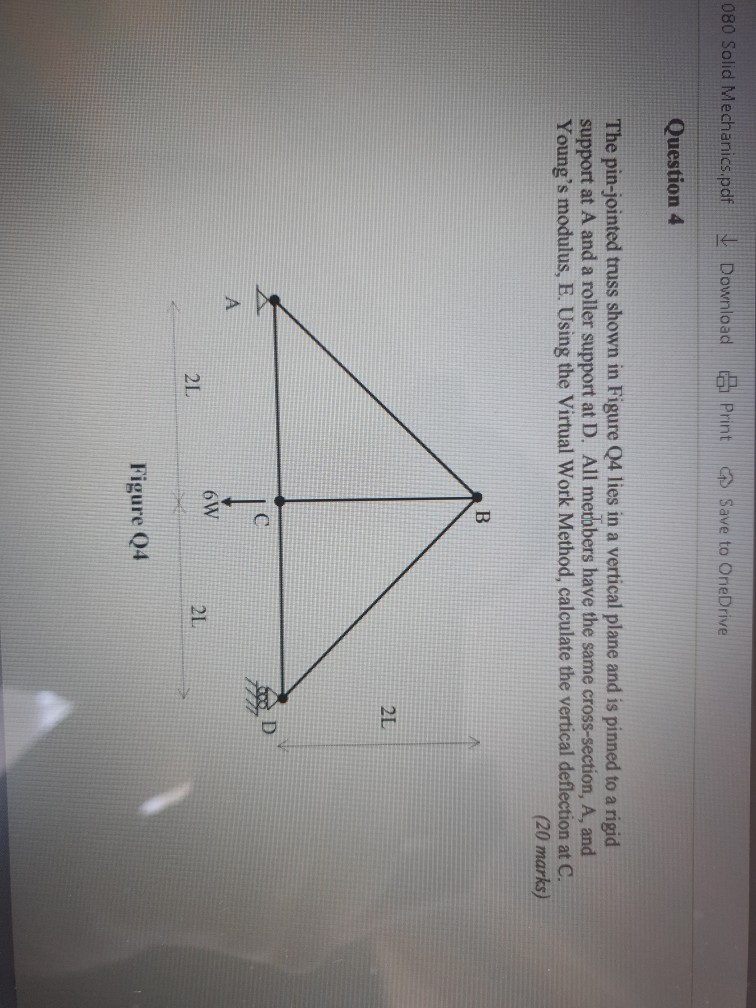080 Solid Mechanics.pdf Download Print > Save to OneDrive Question 4 The pin-jointed truss shown in Figure Q4 lies in a vertical plane and is pinned to a rigid support at A and a roller support at D. All merobers have the same cross-section, A, and Young's modulus, E. Using the Virtual Work Method, calculate the vertical deflection at C. (20 marks) B 2 C D А 6W 2L 21 Figure Q4

• The truss shown in the figure is constructed from three aluminum alloy members, each having a...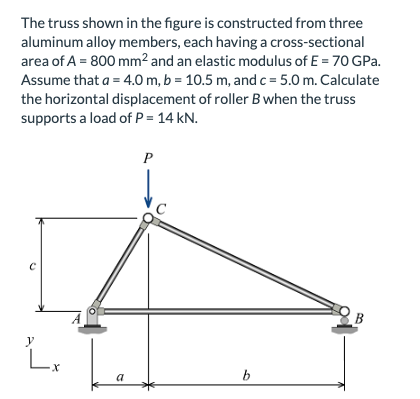The truss shown in the figure is constructed from three aluminum alloy members, each having a cross-sectional area of A 800 mm2 and an elastic modulus of E 70 GPa. Assume that a 4.0 m, b 10.5 m, and c 5.0 m. Calculate the horizontal displacement of roller Bwhen the truss supports a load of P 14 kN B X a The truss shown in the figure is constructed from three aluminum alloy members, each having a cross-sectional area of...